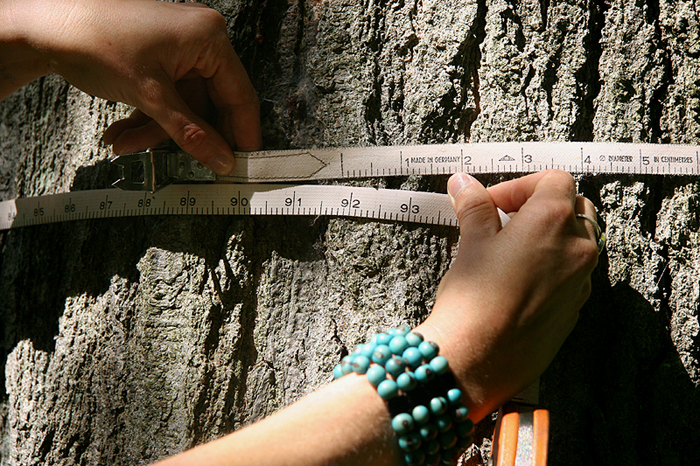# V=B0+B1*D^B2+B3*D^B4*H^B5V=B0+B1*D^B2+B3*D^B4*H^B5

Royal Botanical Garden, Hamilton, Canada 2008

The work is based on a simple mathematical formula used in forestry to calculate the total cubic volume of wood in a tree by measuring its girth and height whilst it is still standing. Using the formula the science and conservation team at RBG calculated the total volume of a Red Oak, killed by Gypsy Moth, before it was cut down. The resulting work uses the entire tree from the largest to smallest sections, cut into cubes and packed as a solid volume. Located where it was felled the work is as much about the space left in the canopy above as it is about the wooden mass.

The work was a direct development from Raw, a previous work commissioned by the Forest of Dean Sculpture Trail